• Slides: 21Linear Lists – Linked List Representation CSE, POSTECH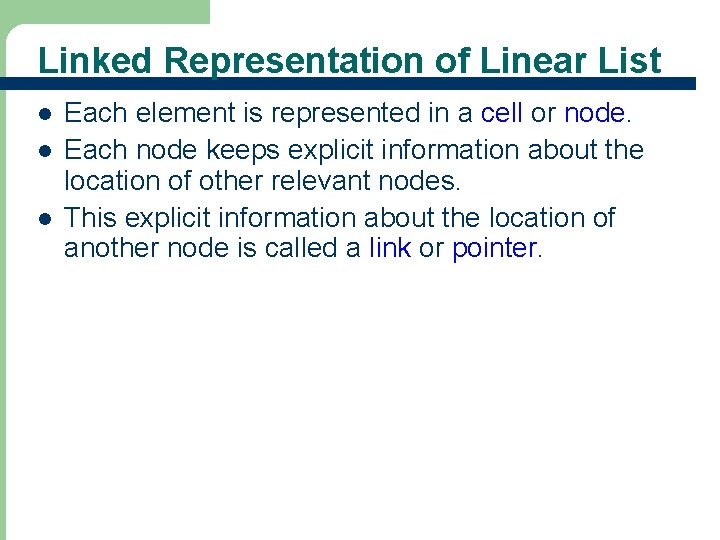Linked Representation of Linear List l l l 2 Each element is represented in a cell or node. Each node keeps explicit information about the location of other relevant nodes. This explicit information about the location of another node is called a link or pointer.Singly Linked List l Let L = (e 1, e 2, …, en) – – – l Each element ei is represented in a separate node Each node has exactly one link field that is used to locate the next element in the linear list The last node, en, has no node to link to and so its link field is NULL. This structure is also called a chain. first Link Field Data Field 3 …… e 1 e 2 e 3 0 enClass ‘Chain. Node’ template <class T> class Chain. Node { friend Chain<T>; private: T data; Chain. Node<T> *link; }; l 4 Since Chain<T> is a friend of Chain. Node<T>, Chain<T> has access to all members (including private members) of Chain. Node<T>.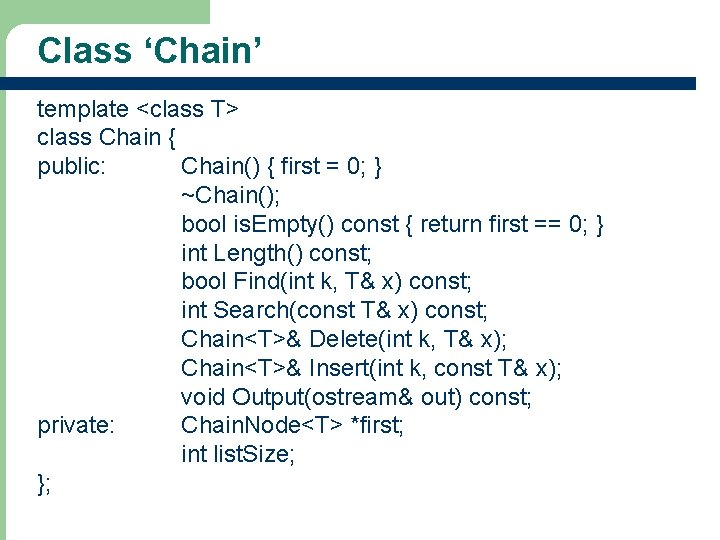Class ‘Chain’ template <class T> class Chain { public: Chain() { first = 0; } ~Chain(); bool is. Empty() const { return first == 0; } int Length() const; bool Find(int k, T& x) const; int Search(const T& x) const; Chain<T>& Delete(int k, T& x); Chain<T>& Insert(int k, const T& x); void Output(ostream& out) const; private: Chain. Node<T> *first; int list. Size; }; 5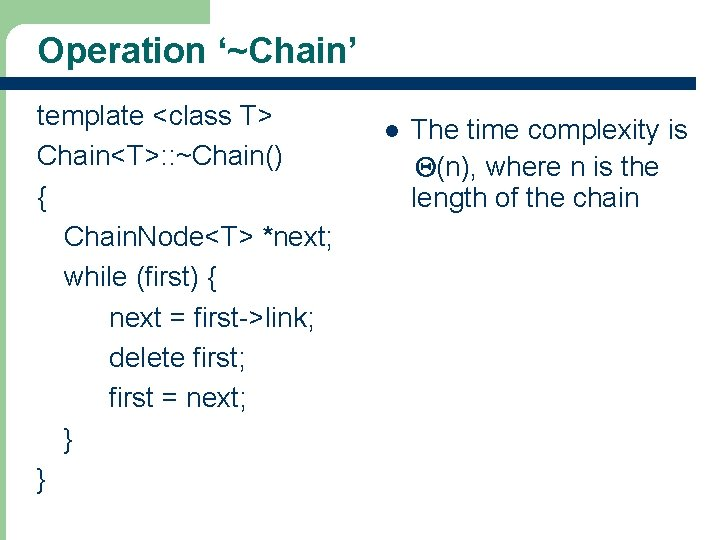Operation ‘~Chain’ template <class T> Chain<T>: : ~Chain() { Chain. Node<T> *next; while (first) { next = first->link; delete first; first = next; } } 6 l The time complexity is Q(n), where n is the length of the chain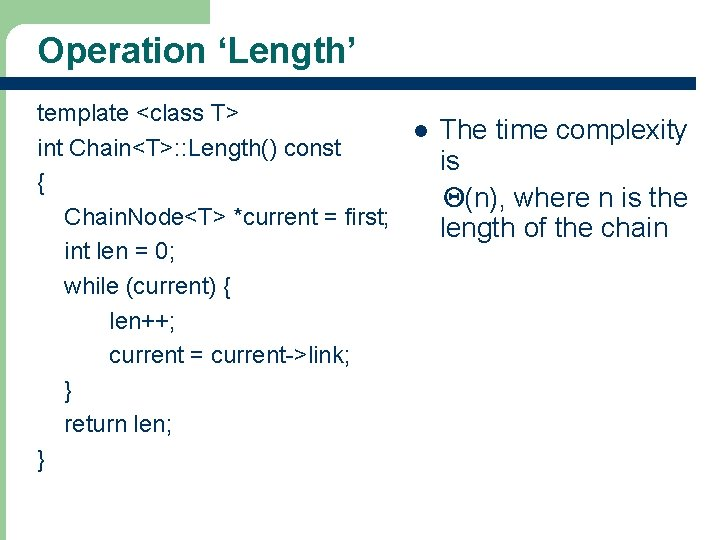Operation ‘Length’ template <class T> l The time complexity int Chain<T>: : Length() const is { Q(n), where n is the Chain. Node<T> *current = first; length of the chain int len = 0; while (current) { len++; current = current->link; } return len; } 7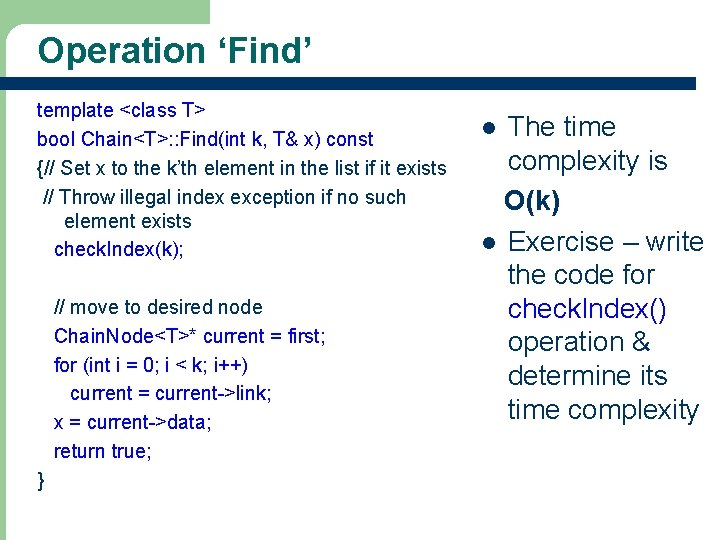Operation ‘Find’ template <class T> bool Chain<T>: : Find(int k, T& x) const {// Set x to the k’th element in the list if it exists // Throw illegal index exception if no such element exists check. Index(k); // move to desired node Chain. Node<T>* current = first; for (int i = 0; i < k; i++) current = current->link; x = current->data; return true; } 8 The time complexity is O(k) l Exercise – write the code for check. Index() operation & determine its time complexity l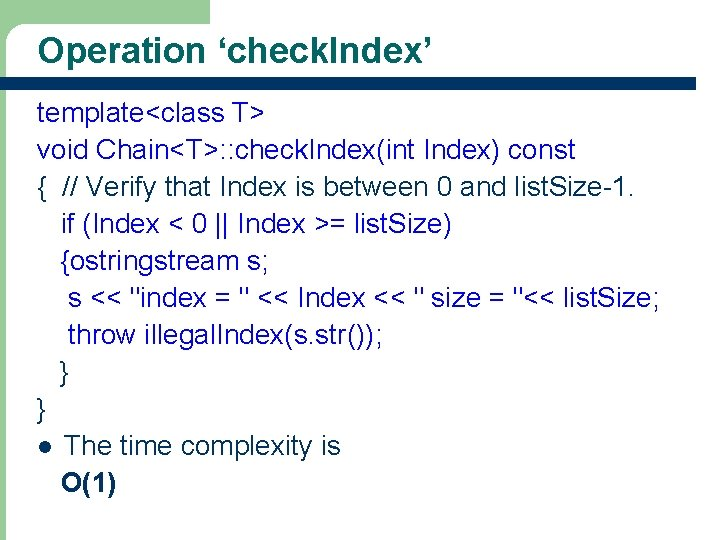Operation ‘check. Index’ template<class T> void Chain<T>: : check. Index(int Index) const { // Verify that Index is between 0 and list. Size-1. if (Index < 0 || Index >= list. Size) {ostringstream s; s << "index = " << Index << " size = "<< list. Size; throw illegal. Index(s. str()); } } l The time complexity is O(1) 9Operation ‘Search’ template <class T> int Chain<T>: : Search(const T& x) const {// Locate x and return its position if found else return -1 // search the chain for x Chain. Node<T>* current = first; int index = 0; // index of current while (current != NULL && current->data != x) { // move to next node current = current->link; index++; } // make sure we found matching element if (current == NULL) return -1; else return index; 10} l The time complexity is O(n)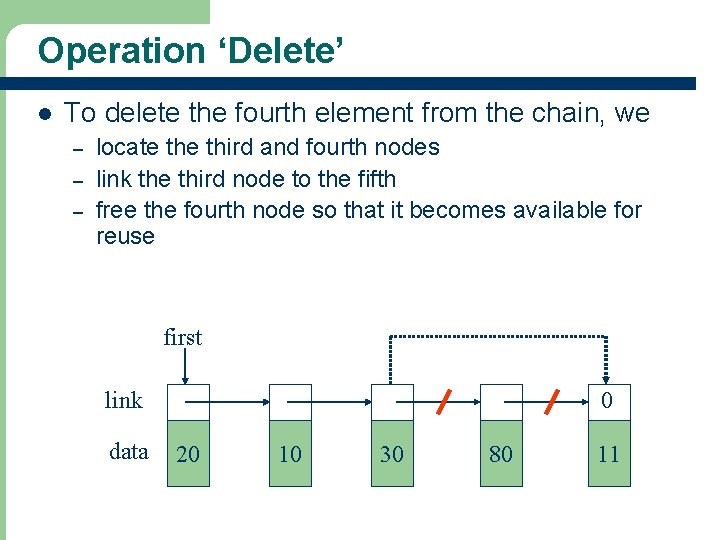Operation ‘Delete’ l To delete the fourth element from the chain, we – – – locate third and fourth nodes link the third node to the fifth free the fourth node so that it becomes available for reuse first link data 11 0 20 10 30 80 11Operation ‘Delete’ l l 12 See Program 6. 7 for deleting a node from a chain The time complexity is O(the. Index)Operation ‘Insert’ l To insert an element following the kth in chain, we – – – locate the kth node new node’s link points to kth node’s link now points to the new node first … … kth node 13 k=0 k>0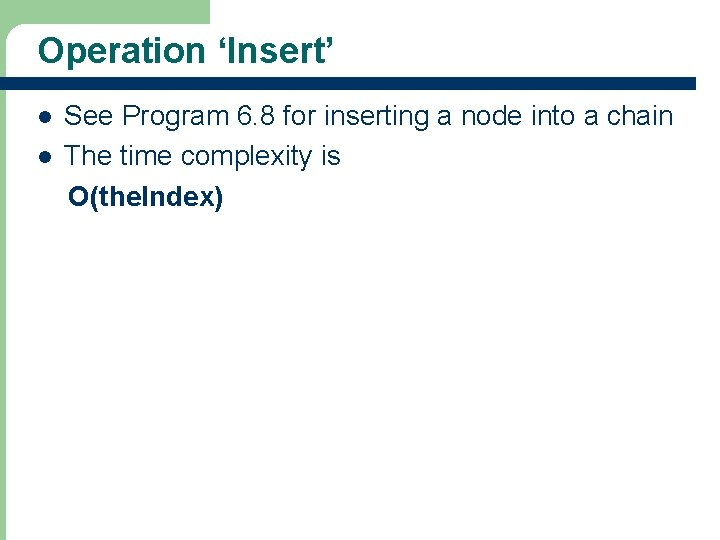Operation ‘Insert’ l l 14 See Program 6. 8 for inserting a node into a chain The time complexity is O(the. Index)Circular List Representation l Programs that use chains can be simplified or run faster by doing one or both of the following: 1. 2. 15 Represent the linear list as a singly linked circular list (or simply circular list) rather than as a chain Add an additional node, called the head node, at the front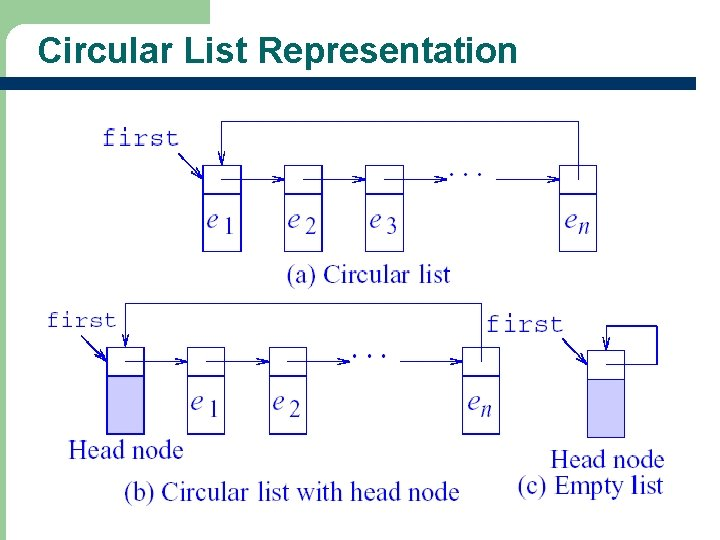Circular List Representation 16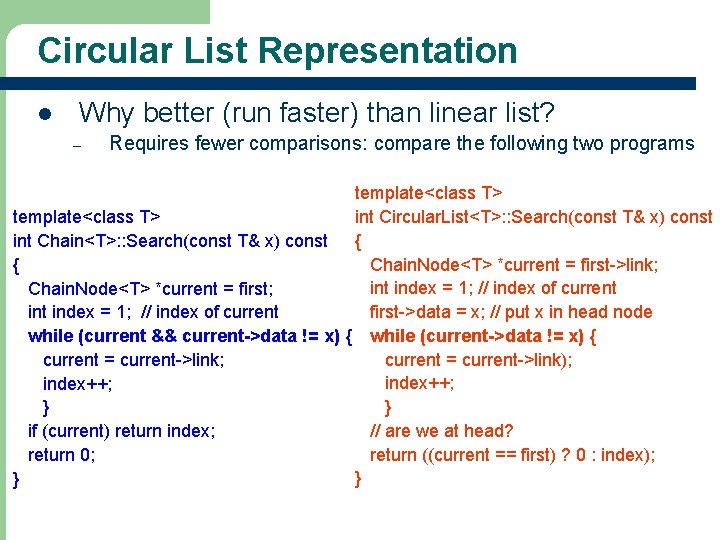Circular List Representation l Why better (run faster) than linear list? – Requires fewer comparisons: compare the following two programs template<class T> int Circular. List<T>: : Search(const T& x) const template<class T> { int Chain<T>: : Search(const T& x) const Chain. Node<T> *current = first->link; { int index = 1; // index of current Chain. Node<T> *current = first; first->data = x; // put x in head node int index = 1; // index of current while (current && current->data != x) { while (current->data != x) { current = current->link); current = current->link; index++; } } // are we at head? if (current) return index; return ((current == first) ? 0 : index); return 0; } } 17Doubly Linked List Representation l 18 An ordered sequence of nodes in which each node has two pointers: left and right.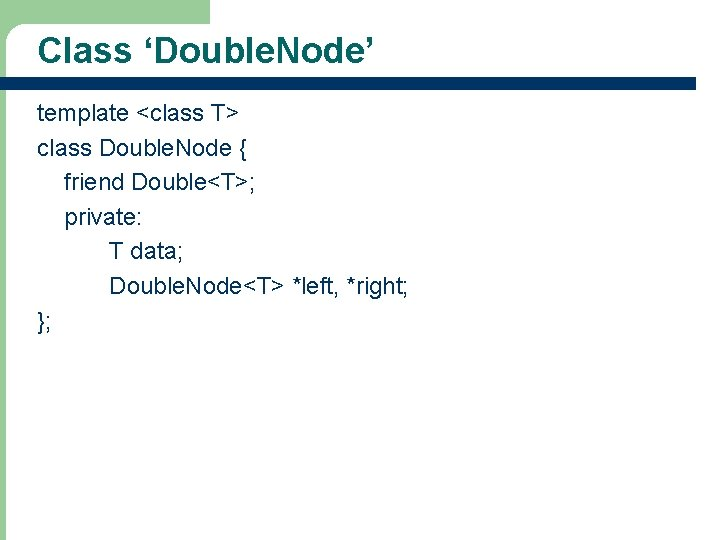Class ‘Double. Node’ template <class T> class Double. Node { friend Double<T>; private: T data; Double. Node<T> *left, *right; }; 19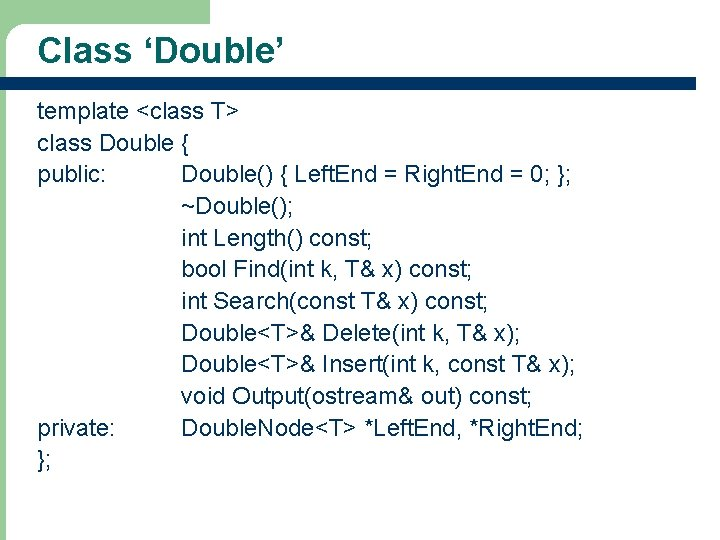Class ‘Double’ template <class T> class Double { public: Double() { Left. End = Right. End = 0; }; ~Double(); int Length() const; bool Find(int k, T& x) const; int Search(const T& x) const; Double<T>& Delete(int k, T& x); Double<T>& Insert(int k, const T& x); void Output(ostream& out) const; private: Double. Node<T> *Left. End, *Right. End; }; 20Circular Doubly Linked List l l Add a head node at the left and/or right ends In a non-empty circular doubly linked list: – – Left. End->left is a pointer to the right-most node (i. e. , it equals Right. End) Right. End->right is a pointer to the left-most node (i. e. , it equals Left. End) l Can you draw a circular doubly linked list with a head at the left end only by modifying Figure 6. 7? l READ Sections 6. 1~6. 4 21